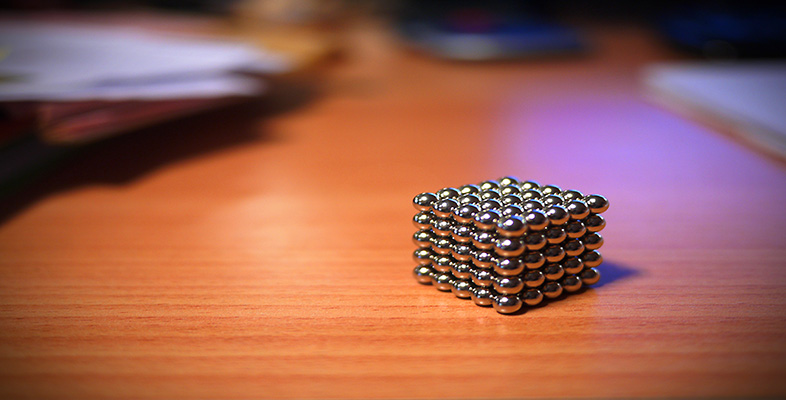Squares, roots and powers

This free course is available to start right now. Review the full course description and key learning outcomes and create an account and enrol if you want a free statement of participation.

Free course

2.1.1 Try some yourself

Activity 15

The size of a population of micro-organisms doubles every hour. If there are two of these creatures to start with, how many will there be after five hours?

After one hour there are 2 × 2 = 4 micro-organisms.

After two hours there are 2 × 4 = 8, or 2 × 2 × 2 = 23.After five hours there are 2 × 32 = 64, or 2 × 2 × 2 × 2 × 2 × 2 = 26.

So there will be 26 = 64 after five hours.

MU120_4M4